Levels »

# But Wait!— That’s Not All!

### The Hole, Elevation: -327.2m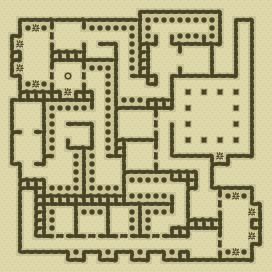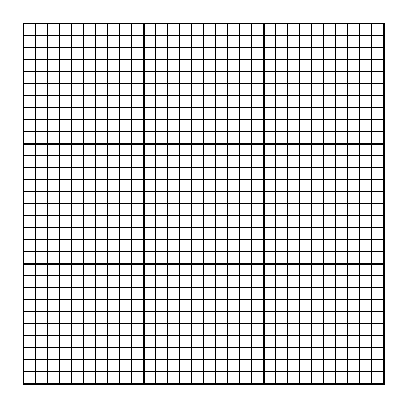### Items

•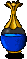2 Clear Blue Potions (15, 17) - (23, 25)
•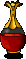2 Bubbling Red Potions (13, 19) - (15, 22)
•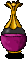Pale Violet Potion (24, 2)

### Monsters

•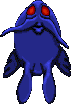12 Greater Nightmares (Random rate: 2) (19, 2) - (24, 2) - (10, 9) - (15, 9) - (25, 13) - (16, 15) - (9, 16) - (16, 17) - (13, 19) - (16, 22) - (6, 25) - (2, 29)
•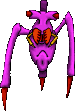48 Venemous Skitters (18, 2) - (23, 2) - (14, 4) - (12, 5) - (20, 5) - (20, 7) - (16, 9) - (21, 9) - (24, 9) - (27, 9) - (29, 10) - (21, 12) - (29, 13) - (15, 14) - (15, 14) - (17, 14) - (15, 15) - (17, 15) - (21, 15) - (8, 16) - (17, 17) - (21, 17) - (25, 17) - (27, 17) - (29, 17) - (15, 18) - (16, 18) - (2, 19) - (6, 19) - (12, 19) - (15, 19) - (16, 19) - (2, 20) - (3, 20) - (4, 20) - (7, 20) - (12, 20) - (15, 22) - (16, 22) - (19, 22) - (7, 26) - (10, 26) - (14, 26) - (19, 26) - (6, 27) - (9, 27) - (11, 27) - (13, 27)
•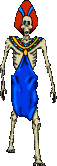Ghasts (Random rate: 6)

### Locations

• 5 Door closers (4, 3) - (4, 8) - (8, 8) - (27, 24) - (27, 29)
• 11 Door openers (6, 3) - (6, 8) - (10, 8) - (19, 14) - (19, 18) - (25, 24) - (6, 29) - (10, 29) - (14, 29) - (18, 29) - (25, 29)
• Save rune(7, 8)

### Teleporters

• (3, 2) Teleporter to (28, 30)
• (1, 4) Teleporter to (24, 6)
• (1, 7) Teleporter to (2, 12)
• (3, 9) Teleporter to (28, 23)
• (28, 23) Teleporter to (3, 9)
• (30, 25) Teleporter to (29, 2)
• (30, 28) Teleporter to (2, 23)
• (28, 30) Teleporter to (3, 2)